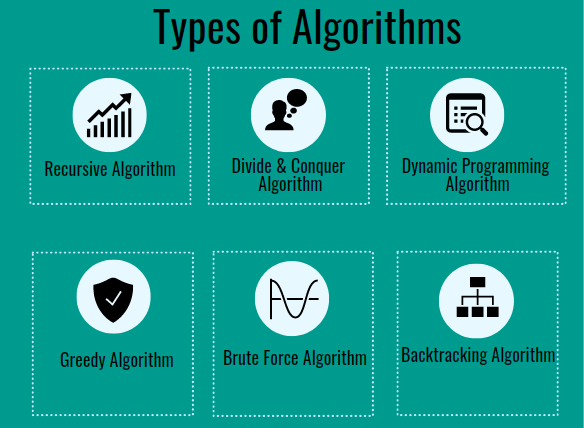Untitled Documenttop of page
Search

# What is an Algorithm?The term “algorithm” does not seem to be relevant to children, but algorithms control everything from the technologies they use to the simple choices they make on a daily basis. Algorithms are interesting, and while some are very complicated, the definition itself is quite straightforward.

A detailed step-by-step instruction, collection, or formula for solving a problem or completing a task is known as an algorithm. In programming, programmers create algorithms that tell the machine how to do something. In other words, an algorithm is a set of well-defined instructions in sequence to solve a problem.

Algorithms are everywhere as you think about them in the truest sense (not just in terms of computing). A formula for cooking is an algorithm, as is the form you use to solve addition or long division problems. Folding a shirt or a pair of trousers is also an algorithm. Even your morning routine can be thought of as an algorithm!

## How Do Algorithms Work At a Basic Level?

The aim of algorithms and machine learning on the internet is to effectively mimic the human brain’s decision-making processes — without us needing to take in all of the knowledge and time required to work through it on our own.

It’s almost as if it’s a shortcut to the human thought process!

Algorithms are, at their most simple stage, a series of if-then statements that run in a program very rapidly to produce a result: If you do A, you can get B. If you want C instead of D, you will get D.

“Where are you from?” you may inquire of a friend.

Certain choices will be your easiest responses depending on their reaction.

You’ll react differently if they say they’re from your childhood hometown than if they say they’re from a city you’ve never been. However, each step leads in a certain direction, and the program follows the workflow or formula to the next stage.The same thing happens when you use Google to look for something.

You can search for “Domino’s pizza” and Google can respond with a recipe or a list of restaurants that serve Domino’s pizzas nearby you; based on the knowledge Google has about you, your location, and your search history.

## Types of Algorithms

Algorithms come in a variety of forms, but the following are the most common:## 1. Recursive algorithm

• This is a fascinating algorithm because it calls itself with a smaller value as inputs, which it obtains after solving for the existing inputs. To put it another way, Recursive Algorithm keeps calling itself recursively before the problem is solved. And hence the name!

• These Algorithms can quickly solve problems like the Tower of Hanoi or the Depth First Search (DFS) of a Graph.

## 2. Divide and Conquer

• Divide and Conquer Algorithms are one of the important methods for resolving a variety of issues. Divide the algorithm into two parts in Divide and Conquer algorithms; the first section divides the problem at hand into smaller sub-problems of the same kind. Then, in the second section, these smaller problems are solved and then combined to produce the final solution to the problem.

• Merge sorting, and quick sorting can be done with divide and conquer algorithms.

## 3. Dynamic Programming Algorithm

• These algorithms work by recalling previous run results and using them to generate new ones. In other words, a Dynamic Programming Algorithm breaks down complex problems into several simple sub-problems, solves each one once, and then saves the results for later use.

• Fibonacci sequence is a good example of Dynamic Programming algorithm.

## 4. Greedy Algorithm

• Greedy Algorithms are used to solve problems involving optimization. We find a locally optimal solution (without respect for potential consequences) and expect to find the optimal solution at the global level using this algorithm.

• The Greedy algorithm is used in Huffman Coding and Dijkstra’s algorithm.

## 5. Brute Force Algorithm

• One of the simplest algorithms in the definition is this one. A Brute Force Algorithm searches all possible solutions randomly in order to find one or more solutions that could solve a function. Consider brute force as attempting to open a safe with any available combinations of numbers.

• E.g. Sequential search

## 6. Backtracking Algorithm

• Backtracking is a method for finding a solution to a problem in a step-by-step manner. It solves problems in a recursive manner, attempting to solve a problem one piece at a time. If one of the options fails, we discard it and go back to the drawing board to try another.

• The N Queens problem is a clear example of how to use the Backtracking algorithm. The N Queen Problem notes that there are N pieces of queens on a chessboard, and we must arrange them in such a way that no queen can strike another queen on the board until it is ordered

## Benefits of Algortihmic Thinking

In subjects like math and science, algorithmic thinking, or the ability to determine simple steps to solve a problem, is critical. Kids, particularly in math, use algorithms without understanding it all the time.

In order to solve a long division puzzle, students use a trained algorithm to iterate over the digits of the number they’re dividing. The child must divide, multiply, and subtract each digit of the dividend (the number being divided). Algorithmic thought enables children to deconstruct problems and formulate ideas in terms of sequential measures in a process. All the more, algorithms are the first step towards becoming an efficient programmer!

Want to strengthen your algorithmic thinking skills and code your way out? Come on then. Let’s break down things into logical steps and work on them one at a time at Rancho labs!